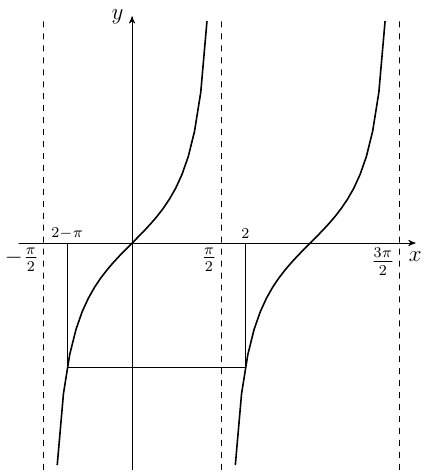# Prove that a function does not have a fixed point

#### Amer

##### Active member
it is a question in my book said

Prove that the function $$f(x) = 2 + x - \tan ^{-1} x$$ has the property $$\mid f'(x)\mid < 1$$
Prove that f dose not have a fixed point

but i found that this function has a fixed point

$$y = 2 + y - \tan ^{-1} y$$

$$y = \tan 2$$

is it right that the question is wrong

Last edited by a moderator:

#### Evgeny.Makarov

##### Well-known member
MHB Math Scholar
Re: Prove that a function dose not have a fixed point

Hint: $\tan^{-1}(\tan 2)\ne 2$.

•Amer

#### Amer

##### Active member
Re: Prove that a function dose not have a fixed point

Hint: $\tan^{-1}(\tan 2)\ne 2$.Since tan(x) is periodic, for each y (such as y = tan(2)) there exists an infinite number of x such that tan(x) = y. Therefore, a convention is needed to select a single value for $\tan^{-1}(y)$. By definition, arctangent returns values in the interval $(-\pi/2,\pi/2)$, called principal values. Therefore, $\tan^{-1}(\tan(2))=2-\pi$.